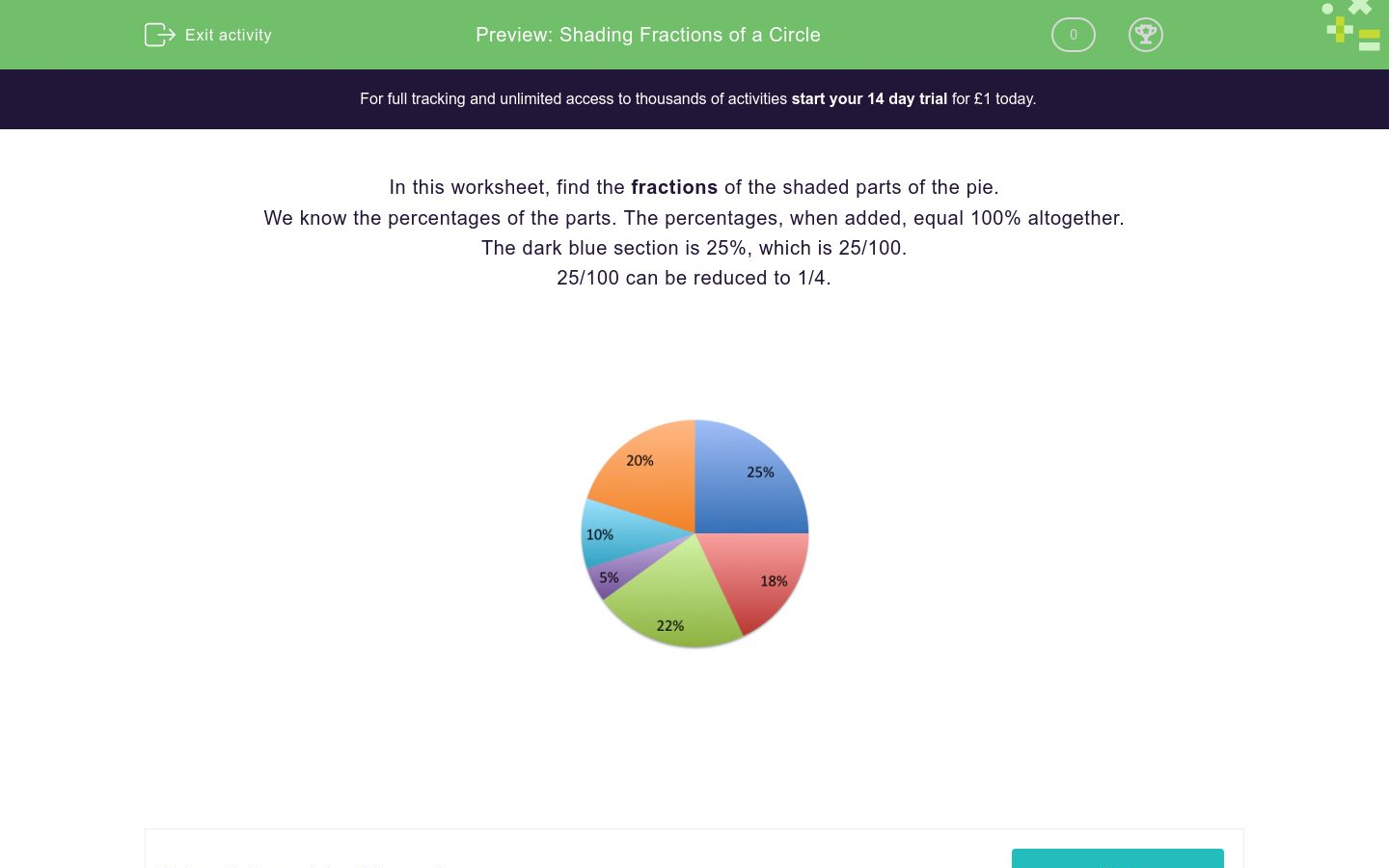# Shading Fractions of a Circle

In this worksheet, students estimate the fraction/amount of a pie chart that is shaded red.Key stage:  KS 2

Curriculum topic:   Ratio and Proportion

Curriculum subtopic:   Solve Shape Problems

Difficulty level:### QUESTION 1 of 10

In this worksheet, find the fractions of the shaded parts of the pie.

We know the percentages of the parts. The percentages, when added, equal 100% altogether.

The dark blue section is 25%, which is 25/100.

25/100 can be reduced to 1/4.What fraction of the pie chart is shaded orange?What fraction of the pie chart is shaded light blue?What fraction of the pie chart is shaded purple?What fraction of the pie chart is shaded green?What fraction of the pie chart is shaded red?What fraction of the pie chart is shaded green and red?What fraction of the pie chart is shaded orange and dark blue?What fraction of the pie chart is shaded purple and light blue?What fraction of the pie chart is shaded orange, dark blue and red?What fraction of the pie chart is shaded dark blue, red and green?• Question 1

What fraction of the pie chart is shaded orange?1/5
EDDIE SAYS
We know that the orange section is 20% of the pie. 20% = 20/100 = 1/5 So 1/5 of the pie is shaded orange.
• Question 2

What fraction of the pie chart is shaded light blue?1/10
EDDIE SAYS
We know that the light blue section is 10% of the pie. 10% = 10/100 = 1/10 So 1/10 of the pie is shaded light blue.
• Question 3

What fraction of the pie chart is shaded purple?1/20
EDDIE SAYS
We know that the purple section is 5% of the pie. 5% = 5/100 = 1/20 So 1/20 of the pie is shaded purple.
• Question 4

What fraction of the pie chart is shaded green?11/50
EDDIE SAYS
We know that the green section is 22% of the pie. 22% = 22/100 = 11/50 So 11/50 of the pie is shaded green.
• Question 5

What fraction of the pie chart is shaded red?9/50
EDDIE SAYS
We know that the red section is 18% of the pie. 18% = 18/100 = 9/50 So 9/50 of the pie is shaded red.
• Question 6

What fraction of the pie chart is shaded green and red?2/5
EDDIE SAYS
Add the green and red sections together 22% + 18 % = 40% 40% = 40/100 = 2/5 So 2/5 of the pie is shaded green and red.
• Question 7

What fraction of the pie chart is shaded orange and dark blue?9/20
EDDIE SAYS
Add the orange and dark blue sections together 20% + 25% = 45% 45% = 45/100 = 9/20 So 9/20 of the pie is shaded orange and dark blue.
• Question 8

What fraction of the pie chart is shaded purple and light blue?3/20
EDDIE SAYS
Add the purple and light blue sections together 5% + 10% = 15% 15% = 15/100 = 3/20 So 3/20 of the pie is shaded purple and light blue.
• Question 9

What fraction of the pie chart is shaded orange, dark blue and red?63/100
EDDIE SAYS
Add the orange, dark blue and red sections together 20% + 25% + 18% = 63% 63% = 63/100 So 63/100 of the pie is shaded orange, dark blue and red.
• Question 10

What fraction of the pie chart is shaded dark blue, red and green?13/20
EDDIE SAYS
Add the dark blue, red and green sections together 25% + 18% + 22% = 65% 65% = 65/100 = 13/20 So 13/20 of the pie is shaded dark blue, red and green.
---- OR ----

Sign up for a £1 trial so you can track and measure your child's progress on this activity.

### What is EdPlace?

We're your National Curriculum aligned online education content provider helping each child succeed in English, maths and science from year 1 to GCSE. With an EdPlace account you’ll be able to track and measure progress, helping each child achieve their best. We build confidence and attainment by personalising each child’s learning at a level that suits them.

Get started